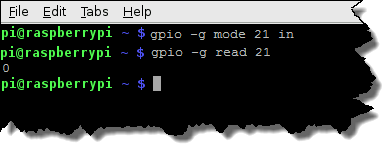The examples in this section show LXTerminal on a Pi running Raspbian.

For a mapping diagram and overlay for your Pi, see Pin-to-Module Mapping.

## Read all GPIO pins

A value of 0 means the I/O point is On; 1 means it’s Off.

Syntax

``````gpio readall
``````

In this image, highlighted GPIO pins are mapped to I/O points.Don’t let the column names in this chart confuse you. In a Readall chart from LXTerminal, the GPIO pin numbers are in the column labeled “BCM.”

## Read a GPIO pinThis example:

• Configures GPIO pin 21 as input.
• Reads the input point mapped to GPIO 21 and writes its value to the terminal.
The input point is at rack position 0, and its status is 0 (On).

Syntax

``````gpio -g mode [GPIO pin number] in
gpio -g read [GPIO pin number]
``````

## Write to outputThis example:

• Configures GPIO pin 20 as output.
• Turns On the output point mapped to the GPIO.
• Reads and writes the point’s value to the terminal.
• Turns Off the point.
• Reads and writes the point’s value.

Syntax

``````gpio -g mode [GPIO pin number] out
gpio -g write [GPIO pin number] 0
gpio -g read [GPIO pin number]
gpio -g write [GPIO pin number] 1
gpio -g read [GPIO pin number]
``````

Back to Examples for Raspberry Pi